### Quadratic Equations Class 10th Mathematics AP Board Solution

##### Class 10th Mathematics AP Board Solution

###### Exercise 5.1
Question 1.

Check whether the following are quadratic equation:

(x + 1)2 = 2(x - 3)

(x + 1)2 = 2(x - 3)

LHS = (x + 1)2 = x2 + 1 + 2x

RHS = 2(x – 3) = 2x – 6

∴ x2 + 1 + 2x = 2x - 6

⇒ x2 + 1 + 2x – 2x + 6 = 0

⇒ x2 + 7 = 0 which is of the form ax2 + bx + c = 0

∴ it is a quadratic equation.

Question 2.

Check whether the following are quadratic equation:

x2 - 2x = ( - 2)(3 - x)

x2 - 2x = ( - 2)(3 - x)

LHS = x2 – 2x

RHS = ( - 2)(3 - x) = - 6 + 2x

∴ x2 - 2x = - 6 + 2x

Or x2 - 2x + 6 – 2x = 0

⇒ x2 + 6 which is of the form ax2 + bx + c = 0

∴ it is a quadratic equation.

Question 3.

Check whether the following are quadratic equation:

(x - 2)(x + 1) = (x - 1) (x + 3)

(x - 2)(x + 1) = (x - 1) (x + 3)

LHS = (x - 2)(x + 1) = x2 + x - 2x - 2 = x2 - x - 2

RHS = (x - 1) (x + 3) = x2 + 3x - x - 3 = x2 + 2x - 3

Equating LHS and RHS

x2 - x - 2 = x2 + 2x - 3

⇒ - 3x + 1 = 0 which is not of the form ax2 + bx + c = 0

Hence it is not a quadratic equation.

Question 4.

Check whether the following are quadratic equation:

(x - 3)(2x + 1) = x(x + 5)

(x - 3)(2x + 1) = x(x + 5)

Here LHS = (x - 3)(2x + 1) = 2x2 - 6x + x - 3 = 2x2 - 5x - 3

RHS = x(x + 5) = x2 + 5x

Equating LHS and RHS

2x2 - 5x - 3 = x2 + 5x

x2 - 10x - 3 = 0 which is of the form ax2 + bx + c = 0

∴ it is a quadratic equation.

Question 5.

Check whether the following are quadratic equation:

(2x - 1) (x - 3) = (x + 5)(x - 1)

(2x - 1) (x - 3) = (x + 5)(x - 1)

Here LHS = (2x – 1)(x - 3) = 2x2 –x - 6x + 3 = 2x2 - 7x + 3

RHS = (x + 5)(x - 1) = x2 + 5x – x - 5 = x2 + 4x - 5

Equating LHS and RHS

2x2 - 7x + 3 = x2 + 4x - 5

x2 – 11x + 8 = 0

which is of the form ax2 + bx + c = 0

∴ it is a quadratic equation.

Question 6.

Check whether the following are quadratic equation:

x2 + 3x + 1 = (x - 2)2

x2 + 3x + 1 = (x - 2)2

Here RHS = (x - 2)2

⇒ x2 + 4 - 4x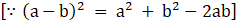Equating LHS and RHS

x2 + 3x + 1 = x2 + 4 - 4x

7x - 3 = 0

Which is not of the form ax2 + bx + c = 0

∴ it is not a quadratic equation.

Question 7.

Check whether the following are quadratic equation:

(x + 2)3 = 2x(x2 - 1)

(x + 2)3 = 2x(x2 - 1)

LHS = (x + 2)3 = x3 + 23 + 3 × x × 2(x + 2)

(∵(a + b)3 = a3 + b3 + 3ab(a + b))

= x3 + 8 + 6x2 + 12x

RHS = 2x3 - 2x

Equating LHS and RHS

x3 + 8 + 6x2 + 12x = 2x3 - 2x

- x3 + 6x2 + 14x + 8 = 0

which is not of the form ax2 + bx + c = 0

∴ it is not a quadratic equation.

Question 8.

Check whether the following are quadratic equation:

x3 - 4x2 - x + 1 = (x - 2)3

x3 - 4x2 - x + 1 = (x - 2)3

RHS = (x - 2)3 = x3 - 23 - 3 × x × 2(x - 2)

(∵(a + b)3 = a3 + b3 + 3ab(a + b))

= x3 - 8 - 6x2 + 12x

Equating LHS and RHS

x3 - 4x2 –x + 1 = x3 - 8 - 6x2 + 12x

2x2 - 13x + 9 = 0

which is of the form ax2 + bx + c = 0

∴ it is a quadratic equation.

Question 9.

Represent the following situations in the form of quadratic equation:

The area of a rectangular plot is 528m2. The length of the plot is one meter more than twice its breadth. We need to find the length and breadth of the plot.

Let length = L and breath = B

Area of rectangle (A) = L × B

According to the given conditions

L = 2B + 1

∴ A = (2B + 1) B

⇒ 528 = 2b2 + b

⇒ 2b2 + b - 528 = 0

Which is a quadratic equation of the form ax2 + bx + c = 0

Question 10.

Represent the following situations in the form of quadratic equation:

The product of two consecutive positive integers is 306. We need to find the integers.

Let us suppose the two consecutive numbers to be x and x + 1

According to the given condition x(x + 1) = 306

x2 + x - 306 = 0, which is the required quadratic equation of the form ax2 + bx + c = 0

Question 11.

Represent the following situations in the form of quadratic equation:
Rohan’s mother is 26 years older than him. The product of their ages after 3 years will be 360 years. We need to find Rohan’s present age.

Let age of Rohan = x

⇒ Rohan’s mother age = x + 26

After 3 years, Rohan’s age = x + 3

and mother’s age = (x + 29) years

According to the given condition

(x + 3) (x + 29) = 360

x2 + 32x - 273 = 0, which is quadratic equation of the form ax2 + bx + c = 0

x2 + 39x - 7x -273 = 0

x(x + 39) -7 (x+ 39) = 0

(x-7)(x+39)= 0
x = 7 or x = -39

but age cannot be negative.

hence, x = 7

Question 12.

Represent the following situations in the form of quadratic equation:

A train travels a distance of 480 km at a uniform speed. If the speed had been 8 km/h less, then it would have taken 3 hours more to cover the same distance. We need to find the speed of the train.

Let the speed of the train be x

Time taken to cover 480 km =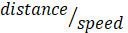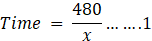If the speed had been 8 km/h less than the train would take 3 hours to cover the same distance

Then speed will be x - 8 and time will be =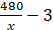Speed =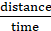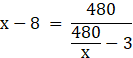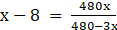⇒ 480x - 3x2 - 3840 + 24x = 480x

⇒ - 3(x2 - 8x + 1280) = 0

⇒ (x2 - 8x + 1280) = 0

which is the required quadratic equation of the form ax2 + bx + c = 0

###### Exercise 5.2
Question 1.

Find the roots of the following quadratic equation by factorization:

x2 - 3x - 10 = 0

⇒ x2 - 5x + 2x - 10 = 0

⇒ x(x - 5) + 2(x - 5) = 0

⇒ (x + 2) (x - 5) = 0

The roots of the equation are those value for which (x + 2) (x - 5) = 0

⇒ x = - 2 or 5

- 2 and 5 are the roots of the given equation.

Question 2.

Find the roots of the following quadratic equation by factorization:

2x2 + x - 6 = 0

2x2 + x - 6 = 0

⇒ 2x2 + 4x - 3x - 6 = 0

⇒ 2x(x + 2) – 3(x + 2) = 0

⇒ (2x - 3)(x + 2) = 0

The roots of the equation are those value for which (x + 2) (2x - 3) = 0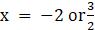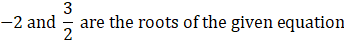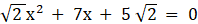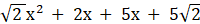= 0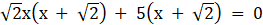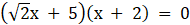The roots of the equation are those value for which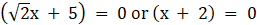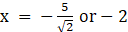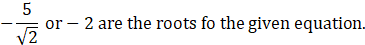Question 4.

Find the roots of the following quadratic equation by factorization: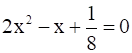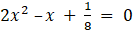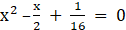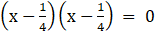The roots of the equation are those value for which⇒ x = 1/4

Hence 1/4 and 1/4 are the roots of the given equation.

Question 5.

Find the roots of the following quadratic equation by factorization:

100x2 - 20x + 1 = 0

100x2 - 20x + 1 = 0

⇒ 100x2 - 10x - 10x + 1 = 0

⇒ 10x(10x - 1) - 1( 10x - 1) = 0

⇒ (10x - 1) (10x - 1) = 0

The roots of the equation are those value for which (10x - 1) (10x - 1) = 0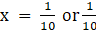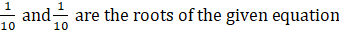.

Question 6.

Find the roots of the following quadratic equation by factorization:

x(x + 4) = 12

x(x + 4) = 12

⇒ x2 + 4x - 12 = 0

⇒ x2 + 6x - 2x - 12 = 0

⇒ x( x + 6) - 2( x + 6) = 0

⇒ (x - 2) (x + 6) = 0

The roots of the equation are those value for which (x - 2) (x + 6) = 0

⇒ x = 2 or - 6

2 and - 6 are the roots of the given equation.

Question 7.

Find the roots of the following quadratic equation by factorization:

3x2 - 5x + 2 = 0

3x2 - 5x + 2 = 0

⇒ 3x2 – 2x - 3x + 2 = 0

⇒ x(3x - 2) - 1(3x - 2) = 0

⇒ (x - 1) (3x - 2) = 0

The roots of the equation are those value for which (x - 1) (3x - 2) = 0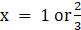Hence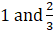are the roots of the given equation.

Question 8.

Find the roots of the following quadratic equation by factorization: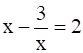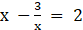⇒ x2 - 2x - 3 = 0

⇒ x2 – 3x + 1x - 3 = 0

⇒ x(x - 3) + 1(x - 3) = 0

⇒ (x + 1) (x - 3) = 0

The roots of the equation are those value for which (x + 1) (x - 3) = 0

⇒ x = - 1 or 3

Hence - 1 and 3 are the roots of the given equation.

Question 9.

Find the roots of the following quadratic equation by factorization:

3(x - 4)2 - 5(x - 4) = 12

3(x - 4)2 - 5(x - 4) = 12

⇒ 3( x2 + 16 - 8x) - 5x + 20 - 12 = 0

⇒ 3x2 + 48 – 24x - 5x + 20 - 12 = 0

⇒ 3x2 – 29x + 56 = 0

⇒ 3x2 – 21x - 8x + 56 = 0

⇒ 3x(x - 7) - 8( x - 7) = 0

⇒ (3x - 8) (x - 7) = 0

The roots of the equation are those value for which (3x - 8) (x - 7) = 0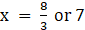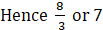are the roots of the given equation.

Question 10.

Find two numbers whose sum is 27 and product is 182.

let the two numbers be x and y

According to the given condition

x + y = 27 ⇒ y = 27 - x

And x × y = 182

⇒ x (27 –x) = 182

⇒ 27x – x2 - 182 = 0

⇒ x2 – 27x + 182 = 0

⇒ x2 – 13x – 14x + 182 = 0

⇒ x (x - 13) - 14 (x - 13) = 0

⇒ (x - 14) (x - 13) = 0

The roots of the equation are those value for which(x - 14) (x - 13) = 0

⇒ x = 14 or 13

Hence two numbers are 14 and 13

Question 11.

Find two consecutive positive integers, sum of whose square is 613.

Let the two consecutive positive integers be x and x + 1

According to the given condition

x2 + (x + 1)2 = 613

⇒ x2 + x2 + 1 + 2x = 613

⇒ 2x2 + 2x – 612 = 0

⇒ x2 + x - 306 = 0

⇒ x2 + 18x – 17x - 306 = 0

⇒ x(x + 18) - 17(x + 18) = 0

⇒ (x + 18)(x - 17) = 0

The roots of the equation are those value for which(x + 18)(x - 17) = 0

⇒ x = 17 or - 18

Hence the two positive integers are 17 and 18 or - 17 and - 18

Question 12.

The altitude of a right triangle is 7 cm less than its base. If the hypotenuse is 13 cm, find the other two sides.

Consider a right angle ΔABC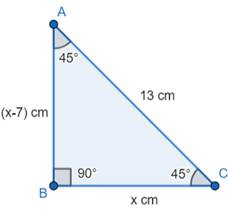Let the base of the Δ be x

⇒ Altitude = x - 7

In the right-angled triangle

According to the Pythagoras theorem

(Hypotenuse)2 = (base)2 + (altitude)2

⇒ 132 = x2 + (x - 7)2

⇒ 169 – x2 – x2 - 49 + 14x = 0

⇒ 2x2 - 14x – 120 = 0

⇒ x2 – 7x - 60 = 0

⇒ x2 – 12x + 5x - 60 = 0

⇒ x( x - 12) + 5( x – 12) = 0

⇒ (x + 5) (x - 12) = 0

⇒ x = - 5 or 12

But base cannot be negative, so x = 12 cm

⇒ altitude = 12 - 7 = 5 cm

Hence 5 cm and 12 cm are the two sides of the given triangle.

Question 13.

A cottage industry produces a certain number of pottery articles in a day. It was observed on a particular day that the cost of production of each article (in rupees) was 3 more than twice the number of articles produced on that day. If the total cost of production on that day was Rs.90 , find the number of articles produced and the cost of each articles.

Let x be the number of articles produced on that day

Thus cost of production of each article = 2x + 3

Total cost = Rs 90

According to the given condition

x(2x + 3) = 90

⇒ 2x2 + 3x - 90 = 0

⇒ 2x2 - 12x + 15x – 90 = 0

⇒ 2x(x - 6) + 15(x - 6) = 0

⇒ (2x + 15) (x - 6) = 0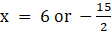Since the number of articles produced cannot be negative ∴ x = 6

And the cost of each article = 2 × 6 + 3 = Rs 15

Number of articles = 6

Cost of each article = 15

Question 14.

Find the dimensions of a rectangle whose perimeter is 28 meters and whose area is 40 square meters.

Let the length and the breath of the rectangle be l and b respectively.

According to the given condition

l × b = 40 m2 ( area = length × breath)…………..1

and

Perimeter = 2(l + b)

2(l + b) = 28

⇒ l + b = 14

⇒ b = 14 –l

Putting value of b in 1

l( 14 - l) = 40

⇒ l2 – 14 l + 40 = 0

⇒ l2 – 10l – 4l + 40 = 0

⇒ l( l - 10) – 4(l - 10) = 0

⇒ (l - 4) ( l - 10) = 0

⇒ l = 4 or 10 m

Hence length is 4 m

And breath is 10 m.

Question 15.

The base of a triangle is 4cm longer than its altitude. If the area of the triangle is 48 sq.cm, the find its base and altitude.

let the base of the triangle be x cm and altitude be y cm

Given base = 4 + y

Area of the triangle = 1/2 × base × altitude

48 = 1/2 × (4 + y) × y

⇒ 96 = 4y + y2

⇒ y2 + 4y – 96 = 0

⇒ y2 + 12y - 8 y – 96 = 0

⇒ y( y + 12) - 8 ( y + 12) = 0

⇒ (y - 8) ( y + 12) = 0

⇒ y = 8 or - 12

Since altitude cannot be negative ∴ y = 8

⇒ base = 4 + 8 = 12 cm

Hence, Base = 12 cm; Altitude = 8 cm

Question 16.

Two trains leave a railway station at the same time. The first train travels towards west and the second train towards north. The first train travels 5 km/hr faster than the second train If after two hours they are 50 km. apart find the average speed of each train.

Let x km /hour be the speed of the first train

Then the speed of the second train is (x - 5) km/hour

Given that they are 50 km apart after 2 hours

As per the given conditions

The first train travels from O to A and

The 2nd train travels from O to B

Also given that AB = 50 Km

Since the OAB is a right angled triangle

∴ By Pythagoras theorem

OA2 + OB2 = AB2………….1

Now distance = speed × time

⇒ OA = x × 2

Also OB = 2(x - 5)

Putting value of OB and OA in 1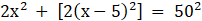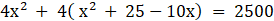⇒ 8 x2 – 40x + 100 = 2500

⇒ x2 – 5x – 300 = 0

⇒ x(x - 20) + 15(x - 20) = 0

⇒ (x + 15) (x - 20) = 0

⇒ x = - 15 or 20

Since speed cannot be negative ∴ the speed of the 1st train = 20 km/hour

And speed of 2nd train is 15 km/hour.

Question 17.

In a class of 60 students, each boy contributed rupees equal to the number of girls and each girl contributed rupees equal to the number of boys. If the total money then collected was D 1600. How many boys are there in the class?

Let the number of girls be x and number of boys be y

Given that the total number of students = 60

⇒ x + y = 60 ⇒ y = 60 –x

Total money collected = 1600

According to the given condition

1600 = xy + yx

⇒ 1600 = 2 xy

⇒ xy = 800

⇒ x( 60 –x) = 800

⇒ x2 - 60x + 800 = 0

⇒ x2 – 20x – 40x + 800 = 0

⇒ x ( x – 20) – 40 (x – 20) = 0

⇒ (x - 40) (x - 20) = 0

⇒ x = 40 or 20

Hence if the number of girls is 40, then the number of boys is 20

And if the number of girls is 20, then the number of boys is 40.

Question 18.

A motor boat heads upstream a distance of 24 km on a river whose current is running at 3 km per hour. The trip up and back takes 6 hours. Assuming that the motor boat maintained a constant speed, what was its speed?

let the speed of the stream be x km/hour

∴ The speed of the boat upstream = (x - 3) km/hr and the speed of the boat downstream = (x + 3)km/hr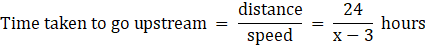Similarly,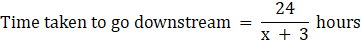According to the given condition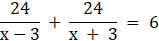⇒ 24(x + 3) + 24 (x - 3) = 6 (x + 3) (x - 3)

⇒ 48x = 6x2 – 54

⇒ x2 - 8x - 9 = 0

⇒ x2 - 9x + x – 9 = 0

⇒ x( x - 9) + (x - 9) = 0

⇒ (x + 1) (x - 9) = 0

⇒ x = - 1 or 9

Since the speed of the stream cannot be negative so x = 9 km/hr

Hence the speed of the stream is 9 km/hr.

###### Exercise 5.3
Question 1.

Find the roots of the following quadratic equation if they exist.

2x2 + x - 4 = 0

2x2 + x - 4 = 0

The equation is of the form ax2 + bx + c = 0

Since a≠0 ,so it exists

We use the quadratic formula to evaluate the roots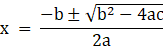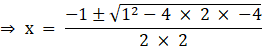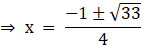The two roots are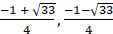Question 2.

Find the roots of the following quadratic equation if they exist.

4x2 + 4√3 + 3 = 0

4x2 + 4√3 + 3 = 0

The equation is of the form ax2 + bx + c = 0

Since a≠0 ,so it exists

We use the quadratic formula to evaluate the roots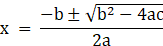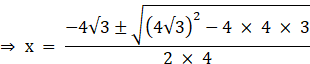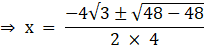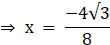The two roots are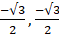Question 3.

Find the roots of the following quadratic equation if they exist.

5x2 - 7x - 6 = 0

5x2 - 7x - 6 = 0

The equation is of the form ax2 + bx + c = 0

Since a≠0 ,so it exists

We use the method of factorization to solve it

⇒ 5x2 - 10x + 3x - 6 = 0

⇒ 5x(x - 2) + 3(x - 2) = 0

⇒ (5x + 3)(x - 2) = 0

⇒ x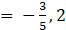Question 4.

Find the roots of the following quadratic equation if they exist.

x2 - 6x + 5 = 0

x2 - 6x + 5 = 0

The equation is of the form ax2 + bx + c = 0

Since a≠0 ,so it exists

We use the method of factorization to solve it

⇒ x2 - 5x - x + 5 = 0

⇒ x(x - 5) - 1(x - 5) = 0

⇒ (x - 1)(x - 5) = 0

⇒ x = 1,5

Question 5.

Find the roots of the quadratic equations given in Q.1 above by applying the quadratic formula.

2x2 + x - 4 = 0

We use the quadratic formula to evaluate the roots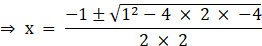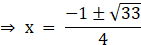The two roots are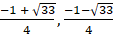Question 6.

Find the roots of the quadratic equations given in Q.1 above by applying the quadratic formula.

4x2 + 4√3 + 3 = 0

We use the quadratic formula to evaluate the rootsThe two roots areQuestion 7.

Find the roots of the quadratic equations given in Q.1 above by applying the quadratic formula.

5x2 - 7x - 6 = 0

We use the method of factorization to solve it

⇒ 5x2 - 10x + 3x - 6 = 0

⇒ 5x(x - 2) + 3(x - 2) = 0

⇒ (5x + 3)(x - 2) = 0

⇒ xQuestion 8.

Find the roots of the quadratic equations given in Q.1 above by applying the quadratic formula.

x2 - 6x + 5 = 0

We use the method of factorization to solve it

⇒ x2 - 5x - x + 5 = 0

⇒ x(x - 5) - 1(x - 5) = 0

⇒ (x - 1)(x - 5) = 0

⇒ x = 1,5

Question 9.

Find the roots of the following equation: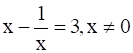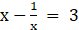⇒ x2 - 3x - 1 = 0

We use the quadratic formula to evaluate the roots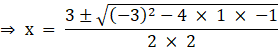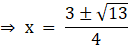The two roots are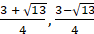Question 10.

Find the roots of the following equation: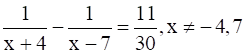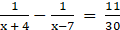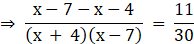⇒ 11(x2 - 3x - 28) = - 330

⇒ x2 - 3x - 28 = - 30

⇒ x2 - 3x + 2 = 0

We use the method of factorization to solve

⇒ x2 - 2x - x + 2 = 0

⇒ x(x - 2) - 1(x - 2) = 0

⇒ (x - 1)(x - 2) = 0

⇒ x = 1, 2

Question 11.

The sum of the reciprocals of Rehman’s ages, (in years) 3 years ago and 5 years from now is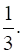Find his present age.

Let Present age of Rehman be x

Three Years ago his age was = x - 3

Five years from now his age will be = x + 5

According to the problem: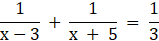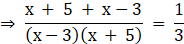⇒ 6(x + 1) = (x - 3)(x + 5)

⇒ 6x + 6 = x2 + 2x - 15

⇒ x2 - 4x - 21 = 0

⇒ x2 - 7x + 3x - 21 = 0

⇒ x(x - 7) + 3(x - 7) = 0

⇒ (x + 3)(x - 7) = 0

⇒ x = - 3 ,7

Since age cannot be negative so ,

Present age is 7 years

Question 12.

In a class test, the sum of Moulika’s marks in Mathematics and English is 30. If she got 2 marks more in Mathematics and 3 marks less in English, the product of her marks have been 210. Find her marks in the two subjects.

Maths = 12, English = 18 (or)

Maths = 13, English = 17

Let marks in Mathematics be x , marks in English be 30 - x

When marks in Mathematics is increased by 2 it becomes x + 2

When marks in English is decreased by 3 it becomes 27 - x

According to the problem the product of the two marks is 210

(x + 2)(27 - x) = 210

⇒ - x2 + 25x + 54 = 210

⇒ x2 - 25x + 156 = 0

Performing factorization we get:

⇒ x2 - 13x - 12x + 156 = 0

⇒ x(x - 13) - 12(x - 13) = 0

⇒ (x - 12)(x - 13) = 0

⇒ x = 12,13

Mathematics = 12 ,English = (30 - 12) = 18

Mathematics = 13, English = (30 - 13) = 17

Question 13.

The diagonal of a rectangular field is 60 metres more than the shorter side. If the longer side is 30 metres more than the shorter side, find the sides of the field.

120 m; 90 m

Let the shorter side be x

Longer side = x + 30

Diagonal = x + 60

Applying Pythagoras theorem

Diagonal2 = Longer side2 + shorter side2

⇒ (x + 60)2 = (x + 30)2 + x2

⇒ x2 + 120x + 3600 = x2 + 60x + 900 + x2

⇒ x2 - 60x - 2700 = 0

Performing factorization we get:

⇒ x2 - 90x + 30x - 2700 = 0

⇒ x(x - 90) + 30(x - 90) = 0

⇒ (x + 30)(x - 90) = 0

⇒ x = - 30, 90

Neglecting the negative term

Shorter Side = 90 m

Longer side = (90 + 30) = 120 m

Question 14.

The difference of squares of two numbers is 180. The square of the smaller number is 8 times the larger number. Find the two numbers.

18, 12; - 18, - 12

Let the larger number be x and smaller number be y

x2 – y2 = 180 …Equation (i)

Since the square of the smaller number is 8 times the larger number, so

⇒ x2 – 8x - 180 = 0

Performing factorization we get:

⇒ x2 - 18x + 10x - 180 = 0

⇒ x(x - 18) + 10(x - 18) = 0

⇒ (x + 10)(x - 18) = 0

x = - 10,18

Rearranging Equation (i)

y2 = x2 - 180

Putting x = - 10 we get

y2 = - 80

Since the square is negative so the solution is neglected

Putting x = 18 we get

y2 = 144

⇒ y =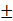12

Question 15.

A train travels 360 km at a uniform speed. If the speed had been 5 km/h more, it would have taken 1 hour less for the same journey. Find the speed of the train.

Let the speed of the train be x

Distance = 360 km

Time taken for a speed of x km/h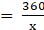When speed is (x + 5) km/h time taken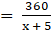According to the problem: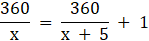⇒ 360(x + 5) = 360x + x(x + 5)

⇒ x2 + 5x - 1800 = 0

Performing factorization we get:

x2 + 45x - 40x - 1800 = 0

⇒ x(x + 45) - 40(x + 45)

⇒ (x - 40)(x + 45) = 0

x = 40, - 45

Since Speed cannot be negative, so

Speed = 40 km/h

Question 16.

Two water taps together can fill a tank in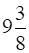hours. The tap of larger diameter takes 10 hours less than the smaller one to fill the tank separately. Find the time in which each tap can separately fill the tank.

Let time taken by the tap of larger diameter be t and smaller diameter be t + 10

Part of tank filled in 1 hour by the tap of larger diameter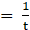Part of tank filled in 1 hour by the tap of smaller diameter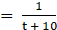Part of tank filled in 1 hour when both the taps were working together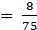So we can say: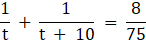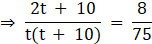⇒ 150t + 750 = 8(t2 + 10t)

⇒ 8t2 - 70t - 750 = 0

⇒ 4t2 - 35t - 375 = 0

Performing factorization we get:

⇒ 4t2 - 60t + 25t - 375 = 0

⇒ 4t(t - 15) + 25(t - 15) = 0

⇒ (4t + 25)(t - 15) = 0

t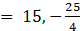Since time is always a positive quantity, so

Time taken by the tap of larger diameter = 15 h

Time taken by the tap of smaller diameter = (15 + 10) = 25 h

Question 17.

An express train takes 1 hour less than a passenger train to travel 132 km between Mysore and Bengaluru (with out taking into consideration the time they stop at intermediate stations). If the average speed of the express train is 11 km/h more than that of the passenger train find the average speed of the two trains.

Let speed of passenger train be x and express train be x + 11

Distance travelled = 132 km

Time difference between the two trains = 1 hours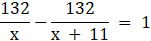⇒ 132x + 1452 - 132x = x(x + 11)

⇒ x2 + 11x - 1452 = 0

Performing factorization we get:

⇒ x2 + 44x - 33x - 1452 = 0

⇒ x(x + 44) - 33(x + 44) = 0

⇒ (x - 33)(x + 44) = 0

⇒ x = 33, - 44

Since speed cannot be negative so

Speed of Passenger train = 33 kmph

Speed of Express Train = (33 + 11) = 44 kmph

Question 18.

Sum of the areas of two squares is 468m2. If the difference of their perimeters is 24m, find the sides of the two squares.

Let the side of one square be x, another square be (x + 6)

Sum of Areas = 468 m2

x2 + (x + 6)2 = 468

2x2 + 12x - 432 = 0

⇒ x2 + 6x - 216 = 0

Performing factorization we get:

⇒ x2 + 18x - 12x - 216 = 0

⇒ x(x + 18) - 12(x + 18) = 0

⇒ (x - 12)(x + 18) = 0

⇒ x = 12, - 18

Neglecting the negative value , we get

Side of squares = 12 m ,18 m

Question 19.

A ball is thrown vertically upward from the top of a building 96m tall with an initial velocity 80m/second. The distances of the ball from the ground after t seconds is s = 96 + 80t - 4.9t2. After how may seconds does the ball strike the ground.

s = 96 + 80t - 4.9t2

When the ball reaches the ground, s = 0

4.9t2 - 80t - 96 = 0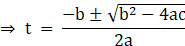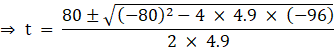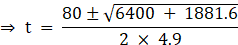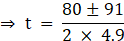Since time cannot be negative so negative root is neglected

⇒ Time taken = 17.44 s

Question 20.

If a polygon of ‘n’ sides has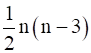diagonals. How many sides will a polygon having 65 diagonals? Is there a polygon with 50 diagonals?

No. of diagonals =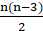n(n - 3) = 2 × 65

⇒ n2 - 3n = 130

⇒ n2 - 3n - 130 = 0

Performing factorization we get:

⇒ n2 - 13n + 10n - 130 = 0

⇒ n(n - 13) + 10(n - 13) = 0

⇒ (n + 10)(n - 13) = 0

n = 13, - 10

Since no. of sides cannot be negative so

No. of Sides = 13

When No. of Diagonals is 50

n(n - 3) = 50 × 2

⇒ n2 - 3n - 150 = 0

Discriminant = (9 - 4 × 1 × ( - 150)) = 609

Since 609 is not a perfect square so n can never be a whole number.

Hence 50 diagonals are not possible

###### Exercise 5.4
Question 1.

Find the nature of the roots of the following quadratic equation. If real roots exist, find them:

2x2 - 3x + 5 = 0

2x2 - 3x + 5 = 0

Discriminant = ( - 3)2 - 4 × 2 × 5 = - 31

Since The Discriminant is negative so the roots are imaginary

Question 2.

Find the nature of the roots of the following quadratic equation. If real roots exist, find them:

3x2 - 4√3 x + 4 = 0

3x2 - 4√3 x + 4 = 0

Discriminant = ( - 4√3)2 - 4 × 3 × 4 = 0

Since The Discriminant is zero so the roots are equal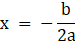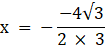The roots are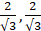Question 3.

Find the nature of the roots of the following quadratic equation. If real roots exist, find them:

2x2 - 6x + 3 = 0

2x2 - 6x + 3 = 0

Discriminant(D) = ( - 6)2 - 4 × 2 × 3 = 12

Since The Discriminant is positive so the roots are real and distinct

The roots are evaluated by using the formula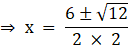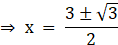The roots are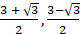Question 4.

Find the value of k for each of the following quadratic equation, so that they have two equal roots.

2x2 + kx + 3 = 0

2x2 + kx + 3 = 0

If the quadratic Equation has equal root then their Discriminant = 0

k2 - 4 × 2 × 3 = 0

⇒ k2 = 24

⇒ k = ±2√6

Question 5.

Find the value of k for each of the following quadratic equation, so that they have two equal roots.

kx(x - 2) + 6 = 0

kx(x - 2) + 6 = 0

⇒ kx2 - 2kx + 6 = 0

If the quadratic Equation has equal root then their Discriminant = 0

4k2 - 4 × k × 6 = 0

⇒ k2 - 6k = 0

⇒ k(k - 6) = 0

k = 0,6

If k = 0 then the equation is no longer Quadratic

Question 6.

Is it possible to design a rectangular mango grove whose length is twice its breadth, and the area is 800m2? If so, find its length and breadth.

Let Breadth be x and length be 2x

Area = (Length × Breadth) = 800m2

2x2 = 800

⇒ x2 = 400

Discriminant = 4 × 1 × 400 = 1600

Hence it is possible

⇒ x = ±20

Neglecting the negative term we have,

Length = 40m

Question 7.

The sum of the ages of two friends is 20 years. Four years ago, the product of their ages in years was 48. Is the above situation possible? If so, determine their present ages.

Let age of one friend be x ,other 20 - x

Four years ago there age was (x - 4) ,(16 - x)

Product of age four years ago = 48

So we can say,

(x - 4)(16 - x) = 48

⇒ x2 - 20x + 112 = 0

Discriminant = (- 20)2 - 4 × 1 × 112 = - 48

Since the discriminant is negative so the roots of the equation are imaginary.

Answer: The above situation is not possible

Question 8.

Is it possible to design a rectangular park of perimeter 80m. and area 400m2? If so, find its length and breadth.

Let Length of rectangular park be x and breadth be (40 - x)

Area = 400 m2

⇒ x(40 - x) = 400

⇒ x2 - 40x + 400 = 0

Discriminant = ( - 40)2 - 4 × 1 × 400

⇒ Discriminant = 1600 - 1600 = 0

Since Discriminant is 0 so it is possible

x2 - 40x + 400 = 0

⇒ (x - 20)2 = 0

⇒ x = 20 m

## PDF FILE TO YOUR EMAIL IMMEDIATELY PURCHASE NOTES & PAPER SOLUTION. @ Rs. 50/- each (GST extra)

SUBJECTS

HINDI ENTIRE PAPER SOLUTION

MARATHI PAPER SOLUTION
SSC MATHS I PAPER SOLUTION
SSC MATHS II PAPER SOLUTION
SSC SCIENCE I PAPER SOLUTION
SSC SCIENCE II PAPER SOLUTION
SSC ENGLISH PAPER SOLUTION
SSC & HSC ENGLISH WRITING SKILL
HSC ACCOUNTS NOTES
HSC OCM NOTES
HSC ECONOMICS NOTES
HSC SECRETARIAL PRACTICE NOTES

2019 Board Paper Solution

HSC ENGLISH SET A 2019 21st February, 2019

HSC ENGLISH SET B 2019 21st February, 2019

HSC ENGLISH SET C 2019 21st February, 2019

HSC ENGLISH SET D 2019 21st February, 2019

SECRETARIAL PRACTICE (S.P) 2019 25th February, 2019

HSC XII PHYSICS 2019 25th February, 2019

CHEMISTRY XII HSC SOLUTION 27th, February, 2019

OCM PAPER SOLUTION 2019 27th, February, 2019

HSC MATHS PAPER SOLUTION COMMERCE, 2nd March, 2019

HSC MATHS PAPER SOLUTION SCIENCE 2nd, March, 2019

SSC ENGLISH STD 10 5TH MARCH, 2019.

HSC XII ACCOUNTS 2019 6th March, 2019

HSC XII BIOLOGY 2019 6TH March, 2019

HSC XII ECONOMICS 9Th March 2019

SSC Maths I March 2019 Solution 10th Standard11th, March, 2019

SSC MATHS II MARCH 2019 SOLUTION 10TH STD.13th March, 2019

SSC SCIENCE I MARCH 2019 SOLUTION 10TH STD. 15th March, 2019.

SSC SCIENCE II MARCH 2019 SOLUTION 10TH STD. 18th March, 2019.

SSC SOCIAL SCIENCE I MARCH 2019 SOLUTION20th March, 2019

SSC SOCIAL SCIENCE II MARCH 2019 SOLUTION, 22nd March, 2019

XII CBSE - BOARD - MARCH - 2019 ENGLISH - QP + SOLUTIONS, 2nd March, 2019

HSC Maharashtra Board Papers 2020

(Std 12th English Medium)

HSC ECONOMICS MARCH 2020

HSC OCM MARCH 2020

HSC ACCOUNTS MARCH 2020

HSC S.P. MARCH 2020

HSC ENGLISH MARCH 2020

HSC HINDI MARCH 2020

HSC MARATHI MARCH 2020

HSC MATHS MARCH 2020

SSC Maharashtra Board Papers 2020

(Std 10th English Medium)

English MARCH 2020

HindI MARCH 2020

Hindi (Composite) MARCH 2020

Marathi MARCH 2020

Mathematics (Paper 1) MARCH 2020

Mathematics (Paper 2) MARCH 2020

Sanskrit MARCH 2020

Important-formula

THANKS